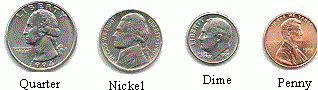Name: ___________________Date:___________________

 Email us to get an instant 20% discount on highly effective K-12 Math & English kwizNET Programs!

### MEAP Preparation - Grade 4 Mathematics1.31 Money Combinations Using Pictures

 1 penny = 1 cent 1 nickel = 5 cents 1 dime = 10 cents 1 quarter = 25 cents 1 dollar = 100 cents 5 pennies = 5 cents 3 dimes = 30 cents Method: Look at the coins Convert each coin to cents or dollar Add the amount Example: How much is this combination worth?Quarter = 25 cents Nickel = 5 cents Dime = 10 cents Penny = 1 cent 25 + 5 + 10 + 1 = 41 cents Answer: 41 cents Directions: Read the questions carefully and fill in the blank for the following questions. Also write at least ten examples of your own.
 Q 1: How much is this combination worth? Answer: _____ centsAnswer: Q 2: How much is this combination worth? Answer: _____ centsAnswer: Question 3: This question is available to subscribers only! Question 4: This question is available to subscribers only!